Compare Fits in Curve Fitting App

Interactive Curve Fitting Workflow

The next topics fit some census data using polynomial equations up to the sixth degree, and a single-term exponential equation. The steps demonstrate how to:

• Load data and explore various fits using different library models.

• Search for the best fit by:

• Comparing graphical fit results

• Comparing numerical fit results including the fitted coefficients and goodness-of-fit statistics

• Export your best fit results to the MATLAB® workspace to analyze the model at the command line.

• Save the session and generate MATLAB code for all fits and plots.

You must load the data variables into the MATLAB workspace before you can fit data using the Curve Fitting app. For this example, the data is stored in the MATLAB file census.mat.

The workspace contains two new variables:

• cdate is a column vector containing the years 1790 to 1990 in 10-year increments.

• pop is a column vector with the U.S. population figures that correspond to the years in cdate.

2. Open the Curve Fitting app:

cftool

3. Select the variable names cdate and pop from the X data and Y data lists.

The Curve Fitting app creates and plots a default fit to X input (or predictor data) and Y output (or response data). The default fit is a linear polynomial fit type. Observe the fit settings display Polynomial, of Degree 1.

4. Change the fit to a second degree polynomial by selecting 2 from the Degree list.

The Curve Fitting app plots the new fit. The Curve Fitting app calculates a new fit when you change fit settings because Auto fit is selected by default. If refitting is time consuming, e.g., for large data sets, you can turn off Auto fit by clearing the check box.

The Curve Fitting app displays results of fitting the census data with a quadratic polynomial in the Results pane, where you can view the library model, fitted coefficients, and goodness-of-fit statistics.

5. Change the Fit name to poly2.

6. Display the residuals by selecting View > Residuals Plot.

The residuals indicate that a better fit might be possible. Therefore, continue exploring various fits to the census data set.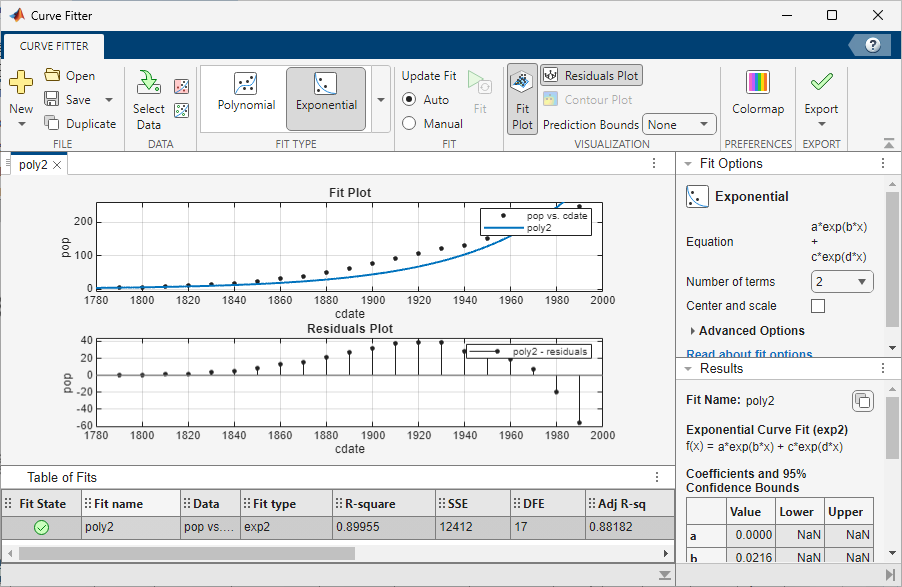7. Add new fits to try the other library equations.

1. Right-click the fit in the Table of Fits and select Duplicate “poly2 (or use the Fit menu).

Tip

For fits of a given type (for example, polynomials), use Duplicate “fitname instead of a new fit because copying a fit requires fewer steps. The duplicated fit contains the same data selections and fit settings.

2. Change the polynomial Degree to 3 and rename the fit poly3.

3. When you fit higher degree polynomials, the Results pane displays this warning:

Equation is badly conditioned. Remove repeated data points
or try centering and scaling.

Normalize the data by selecting the Center and scale check box.

4. Repeat steps a and b to add polynomial fits up to the sixth degree, and then add an exponential fit.

5. For each new fit, look at the Results pane information, and the residuals plot in the Curve Fitting app.

The residuals from a good fit should look random with no apparent pattern. A pattern, such as a tendency for consecutive residuals to have the same sign, can be an indication that a better model exists.

The warning about scaling arises because the fitting procedure uses the cdate values as the basis for a matrix with very large values. The spread of the cdate values results in a scaling problem. To address this problem, you can normalize the cdate data. Normalization scales the predictor data to improve the accuracy of the subsequent numeric computations. A way to normalize cdate is to center it at zero mean and scale it to unit standard deviation. The equivalent code is:

(cdate - mean(cdate))./std(cdate)

Note

Because the predictor data changes after normalizing, the values of the fitted coefficients also change when compared to the original data. However, the functional form of the data and the resulting goodness-of-fit statistics do not change. Additionally, the data is displayed in the Curve Fitting app plots using the original scale.

Determining the Best Fit

To determine the best fit, you should examine both the graphical and numerical fit results.

Examine the Graphical Fit Results

1. Determine the best fit by examining the graphs of the fits and residuals. To view plots for each fit in turn, double-click the fit in the Table of Fits. The graphical fit results indicate that:

• The fits and residuals for the polynomial equations are all similar, making it difficult to choose the best one.

• The fit and residuals for the single-term exponential equation indicate it is a poor fit overall. Therefore, it is a poor choice and you can remove the exponential fit from the candidates for best fit.

2. Examine the behavior of the fits up to the year 2050. The goal of fitting the census data is to extrapolate the best fit to predict future population values.

1. Double-click the sixth-degree polynomial fit in the Table of Fits to view the plots for this fit.

2. Change the axes limits of the plots by selecting Tools > Axes Limits.

3. Alter the X (cdate) Maximum to 2050, and increase the Main Y (pop) Maximum to 400, and press Enter.

4. Examine the fit plot. The behavior of the sixth-degree polynomial fit beyond the data range makes it a poor choice for extrapolation and you can reject this fit.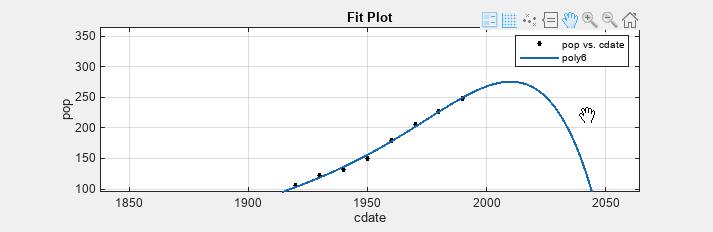Evaluate the Numerical Fit Results

When you can no longer eliminate fits by examining them graphically, you should examine the numerical fit results. The Curve Fitting app displays two types of numerical fit results:

• Goodness-of-fit statistics

• Confidence bounds on the fitted coefficients

The goodness-of-fit statistics help you determine how well the curve fits the data. The confidence bounds on the coefficients determine their accuracy.

Examine the numerical fit results:

1. For each fit, view the goodness-of-fit statistics in the Results pane.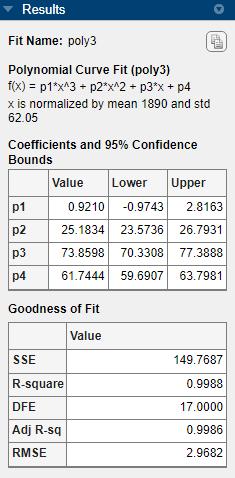2. Compare all fits simultaneously in the Table of Fits. Click the column headings to sort by statistics results.3. Examine the sum of squares due to error (SSE) and the adjusted R-square statistics to help determine the best fit. The SSE statistic is the least-squares error of the fit, with a value closer to zero indicating a better fit. The adjusted R-square statistic is generally the best indicator of the fit quality when you add additional coefficients to your model.

The largest SSE for exp1 indicates it is a poor fit, which you already determined by examining the fit and residuals. The lowest SSE value is associated with poly6. However, the behavior of this fit beyond the data range makes it a poor choice for extrapolation, so you already rejected this fit by examining the plots with new axis limits.

The next best SSE value is associated with the fifth-degree polynomial fit, poly5, suggesting it might be the best fit. However, the SSE and adjusted R-square values for the remaining polynomial fits are all very close to each other. Which one should you choose?

4. Resolve the best fit issue by examining the confidence bounds for the remaining fits in the Results pane. Double-click a fit in the Table of Fits to open (or focus if already open) the fit figure and view the Results pane. A fit figure displays the fit settings, results pane and plots for a single fit.

Display the fifth-degree polynomial and the poly2 fit figures side by side. Examining results side by side can help you assess fits.

1. To show two fit figures simultaneously, use the layout controls at the top right of the Curve Fitting app or select Window > Left/Right Tile or Top/Bottom Tile.

2. To change the displayed fits, click to select a fit figure and then double-click the fit to display in the Table of Fits.

3. Compare the coefficients and bounds (p1, p2, and so on) in the Results pane for both fits, poly5 and poly2. The toolbox calculates 95% confidence bounds on coefficients. The confidence bounds on the coefficients determine their accuracy. Check the equations in the Results pane (f(x)=p1*x+p2*x...) to see the model terms for each coefficient. Note that p2 refers to the p2*x term in Poly2 and the p2*x^4 term in Poly5. Do not compare normalized coefficients directly with non-normalized coefficients.

Tip

Use the View menu to hide the Fit Settings or Table of Fits if you want more space to view and compare plots and results, as shown next. You can also hide the Results pane to show only plots.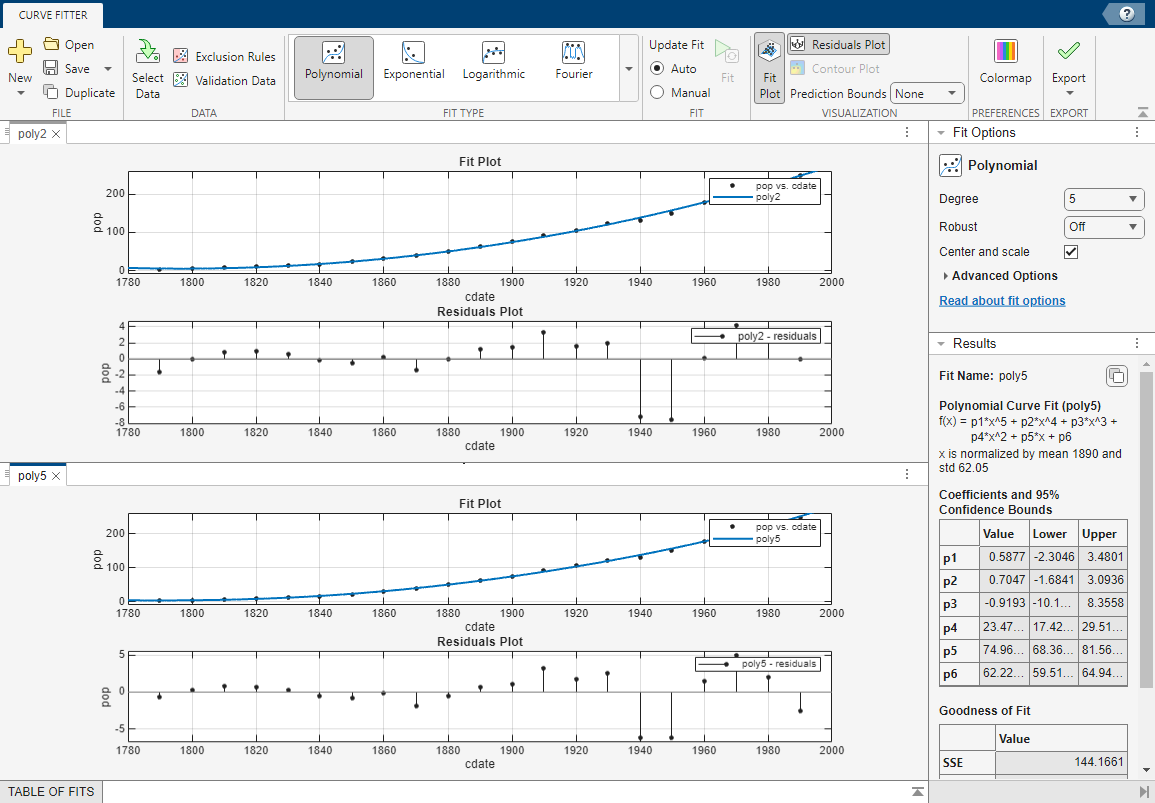The bounds cross zero on the p1, p2, and p3 coefficients for the fifth-degree polynomial. This means you cannot be sure that these coefficients differ from zero. If the higher order model terms may have coefficients of zero, they are not helping with the fit, which suggests that this model overfits the census data.However, the small confidence bounds do not cross zero on p1, p2, and p3 for the quadratic fit, poly2 indicate that the fitted coefficients are known fairly accurately.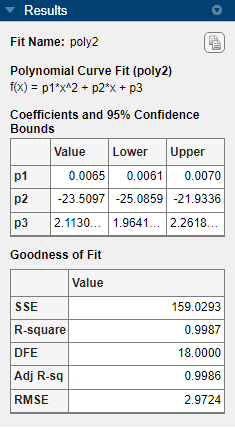Therefore, after examining both the graphical and numerical fit results, you should select poly2 as the best fit to extrapolate the census data.

Note

The fitted coefficients associated with the constant, linear, and quadratic terms are nearly identical for each normalized polynomial equation. However, as the polynomial degree increases, the coefficient bounds associated with the higher degree terms cross zero, which suggests overfitting.

Analyzing Your Best Fit in the Workspace

You can use Save to Workspace to export the selected fit and the associated fit results to the MATLAB workspace. The fit is saved as a MATLAB object and the associated fit results are saved as structures.

1. Right-click the poly2 fit in the Table of Fits and select Save “poly2” to Workspace (or use the Fit menu).2. Click to save with the default names.

The fittedmodel is saved as a Curve Fitting Toolbox™ cfit object.

>> whos fittedmodel
Name             Size            Bytes  Class

fittedmodel      1x1               822  cfit

Examine the fittedmodel cfit object to display the model, the fitted coefficients, and the confidence bounds for the fitted coefficients:

fittedmodel

fittedmodel =
Linear model Poly2:
fittedmodel(x) = p1*x^2 + p2*x + p3
Coefficients (with 95% confidence bounds):
p1 =    0.006541  (0.006124, 0.006958)
p2 =      -23.51  (-25.09, -21.93)
p3 =  2.113e+004  (1.964e+004, 2.262e+004)

Examine the goodness structure to display goodness-of-fit results:

goodness

goodness =
sse: 159.0293
rsquare: 0.9987
dfe: 18
rmse: 2.9724

Examine the output structure to display additional information associated with the fit, such as the residuals:

output

output =
numobs: 21
numparam: 3
residuals: [21x1 double]
Jacobian: [21x3 double]
exitflag: 1
algorithm: 'QR factorization and solve'
iterations: 1

You can evaluate (interpolate or extrapolate), differentiate, or integrate a fit over a specified data range with various postprocessing functions.

For example, to evaluate the fittedmodel at a vector of values to extrapolate to the year 2050, enter:

y = fittedmodel(2000:10:2050)
y =

274.6221
301.8240
330.3341
360.1524
391.2790
423.7137
Plot the fit to the census data and the extrapolated fit values:
plot(fittedmodel, cdate, pop)
hold on
plot(fittedmodel, 2000:10:2050, y)
hold off
For more examples and instructions for interactive and command-line fit analysis, and a list of all postprocessing functions, see Fit Postprocessing.

For an example reproducing this interactive census data analysis using the command line, see Polynomial Curve Fitting.

The toolbox provides several options for saving your work. You can save one or more fits and the associated fit results as variables to the MATLAB workspace. You can then use this saved information for documentation purposes, or to extend your data exploration and analysis. In addition to saving your work to MATLAB workspace variables, you can:

• Save the current curve fitting session by selecting File > Save Session. The session file contains all the fits and variables in your session and remembers your layout. See Save and Reload Sessions.

• Generate MATLAB code to recreate all fits and plots in your session by selecting File > Generate Code. The Curve Fitting app generates code from your session and displays the file in the MATLAB Editor.

You can recreate your fits and plots by calling the file at the command line with your original data as input arguments. You can also call the file with new data, and automate the process of fitting multiple data sets. For more information, see Generating Code from the Curve Fitting App.